## Section12.2The Universal Law of Gravitation

In Principia, Newton presented his three laws of motion, and also stated the law of gravitation. The law of gravitation states that there is an attractive force between all objects that depends on their masses and distances.

The magnitude of the gravitational force $F$ between two particles of masses $m_1$ and $m_2$ separated by a distance $r$ is given by

\begin{equation} F = G_N\, \dfrac{m_1 m_2}{r^2},\label{eq-Newtons-law-of-gravitation}\tag{12.2.1} \end{equation}

where $G_N$ is a universal constant, called gravitational constant. Do not confuse this $G_N$ with acceleration due to gravity $g\text{,}$ although sometimes we write $G$ for $G_N\text{.}$ The value of $G_N$ has been determined experimentally to be

\begin{equation} G_N = 6.67428\times10^{-11}\ \text{N.(m/kg)}^2.\tag{12.2.2} \end{equation}

Constant $G_N$ is considered to be truly universal, being same between any two objects anywhere in the universe, although there has been speculation that its value may have changed over time.

The force is very weak as you can tell if you calculate force between two $1\text{-kg}$ objects $1\text{-m}$ apart. It will be

\begin{equation*} F = 6.67 \times 10^{-11}\text{ N}. \end{equation*}

Experimental verification of the law in a lab experiment was done by Henry Cavendish, who made use of a very sensitive torsion balance, now called the Cavendish balance, to detect small forces. Modern versions of Canvendish experiment are routinely perfomed by advanced physics students.

### Subsection12.2.1Applying the Law of Gravitation to Spherical Bodies

Outside a Spherical Body

Eq. (12.2.1) is for force between point particles. To get the formula for gravitational force between extended bodies, we need to use mathematics to figure out the net force between all particles of the two bodies.

Mathematics (Calculus) shows that the gravitaional force between two spherical bodies that are outside of each other has the same form as Eq. (12.2.1) provided the distance $r$ is center-to-center distance. For instance, the force between Earth and Sun will be

\begin{equation*} F = G_N\, \dfrac{M_{\text{Sun}} M_{\text{Earth}}}{r_{\text{ES}}^2}, \end{equation*}

where $r_{\text{ES}}$ is the distance from the center of the Sun to the center of the Earth.

Similarly, the gravitaitonal force of Earth on a satellite around Earth will be based on the distance from the center of the Earth and the satellite, and not just the altitude of the satellite.

Inside a Spherical Body

But, how about force on an object at a point inside a spherical body? For instance, what will be the force on a miner deep inside the Earth? Math shows that, only the mass of the sphere that is within the distance to the object matters.

Say, an object of mass $m$ inside Earth is at a distance $d$ from the center of Earth. The surprising discovery of the mathematics of spherical bodies is that the force of Earth on such a body will be from only those masses of Earth that are within a distance $d$ from the center. That is, effectively Earth will be of radius $d$ rather than $R_E\text{.}$

\begin{equation*} F = G_N\, \dfrac{m M_d}{d^2}, \end{equation*}

where $M_d$ is the part of Earth that is within a distance $d$ from its center.

Inside a Spherical Shell

One of the consequences of this result is that if you sit inside a spherical shell, the gravitational force on you will be zero no matter where you sit, since there is no mass within your distance from the center.

Evaluate the gravitational force in the following situations:

(a) between two 5-kg spherical steel balls separated by a center-to-center distance of $15\text{ cm}\text{,}$ and,

(b) the Sun and the planet Neptune which have an average separation of $30.15\text{ AU}\text{.}$ $M_\text{Sun} \approx 2.0\times 10^{30}\text{ kg}\text{,}$ $M_\text{Neptune} \approx 1.0\times 10^{26}\text{ kg}$ and $1\text{ AU} = 1.496\times 10^8\text{ km}\text{.}$

Hint

(a) and (b) : Use formulas for spherical bodies.

(a) $7.41\times 10^{-8}\text{ N}\text{,}$ (b) $6.56\times 10^{20}\text{ N}\text{.}$

Solution

(a) We use the formulas given.

\begin{align*} F \amp = G_N \dfrac{m_1 m_2}{r^2},\\ \amp = 6.67\times 10^{-11} \dfrac{ 5\times 5}{0.15^2} = 7.41\times 10^{-8}\text{ N}. \end{align*}

(b) We use the formulas given.

\begin{align*} F \amp = G_N \dfrac{m_1 m_2}{r^2},\\ \amp = 6.67\times 10^{-11} \dfrac{2.0\times 10^{30}\times 1.0\times 10^{26}}{(30.15\times 1.496\times 10^8\times 10^3)^2}, \\ \amp = 6.56\times 10^{20}\text{ N}. \end{align*}

### Subsection12.2.2Applying the Law of Gravitation to Arbitrary Bodies

If the distance between two bodies is large compared to their sizes, the bodies can be replaced by point masses and then we can use the same form of the law as given in Eq. (12.2.1) for point particles. The force of attraction between non-spherical objects which are not far apart compared to their sizes is complicated and can only be computed by dividing the bodies into smaller parts and vectorially summing up the forces between every pair of masses.

It is interesting to note that a straightforward analysis of the falling of an apple and the continuous falling of the Moon towards the Earth can lead one to the discovery of the inverse distance square part of the universal law of gravitation. Although, Newton did not come upon the universal law of gravitation in this way, the analysis is none-the-less instructive, and will be presented here.

From the observations on freely falling objects near the Earth, you know that the apple falls with an acceleration of $9.81\text{ m/s}^2\text{.}$ From observations on the Moon, i.e. from the distance to the Moon and its time period, it is also known that the Moon has a centripetal acceleration of $0.00272\text{ m/s}^2$ in its circular orbit about Earth.

Thus, the acceleration of the Moon is approximately 3600 times less than that of apple.

\begin{equation*} \dfrac{a_{\text{Moon}}}{a_{\text{apple}}} = \dfrac{0.00272\ \text{m/s}^2}{9.81\ \text{m/s}^2} \approx \dfrac{1}{3600} \end{equation*}

Since Moon is at a distance of approximately $60$ times the radius of Earth, it can be concluded that the acceleration due to the gravitation of the Earth drops as the inverse of the square of the distance.

\begin{equation*} \dfrac{a_{\text{Moon}}}{a_{\text{apple}}} = \dfrac{r_{\text{apple}}^2}{r_{\text{Moon}}^2} \end{equation*}

Since, force on an object is proportional to the acceleration, the force must also vary as $1/r^2$ if the acceleration varies as such. The genius of Newton was in the realization that the same law of force must apply between Earth and Moon as applies between Earth and apple.

\begin{equation*} \dfrac{F}{m}\propto \dfrac{1}{r^2} \end{equation*}

Here $m$ may stand for the mass of the apple or the Moon.

Find gravitational forces between objects in the following situations.

(a) Two spherical lead balls of masses 10 kg each separated by center-to-center distance of 10 cm.

(b) Two people of height approximately 1.5 m and masses 100 kg and 60 kg separated by a distance of 2 m.

(c) Same two people 20 m apart.

(d) A satellite of mass 1000 kg in orbit 200 km above the Earth; use mass of the Earth = $5.96\times 10^{24}\text{ kg}\text{,}$ and mean radius of the Earth = $6.37\times 10^6\text{ m}\text{.}$

Hint

(a) force same as point objects, (b) Too complicated to calculate, (c) approximately same as point objects, (d) same as a spherical object and a point object outside of the sphere.

(a) $6.67\times 10^{-7}\ \textrm{N} \text{,}$ (b) Crude idea $1.0\times 10^{-8}\ \textrm{N} \text{,}$ (c) $1.0\times 10^{-10}\ \textrm{N} \text{,}$ (d) $9.2\times 10^{3}\ \textrm{N}\text{.}$

Solution

(a) I have stated above that a spherical object “acts” same as a point particle as far as gravitation outside the sphere is concerned. Therefore, the force between the spherical lead balls can be easily computed giving the following magnitude.

\begin{equation*} F = \left(6.67\times 10^{-11} \right) \times \dfrac{10\ \times 10\ }{\left( 0.1\ \right)^2} = 6.67\times 10^{-7}\ \textrm{N}. \end{equation*}

(b) Since people are not spherical objects, and since their separation is not too great compared to their sizes, we cannot use the same form of the law of gravitation as given for the point particles. If we use the formula given, we will only get some rough estimate, not an exact answer for the magnitude of the force.

\begin{equation*} F(\text{CRUDE IDEA}) = \left(6.67\times 10^{-11} \right) \times \dfrac{100\ \times 100\ }{\left( 2\ \textrm{m}\right)^2} = 1.0\times 10^{-8}\ \textrm{N}. \end{equation*}

(c) In this part, the people are separated by more than 10-times their sizes. Therefore approximating them as spheres will not give us too different a result than an exact result. Although the result of spherical approximation for a person will not give an exact result, but we expect the result to be more precise here than it was in part (b). The magnitude of the force is

\begin{equation*} F \approx \left(6.67\times 10^{-11} \right) \times \dfrac{100\ \times 100\ }{\left( 20\ \right)^2} = 1.0\times 10^{-10}\ \textrm{N}. \end{equation*}

(d) Although, the satellite is not a spherical object, it is far away from the Earth compared to the size of the satellite. Therefore, we can treat the satellite as a point mass. This gives the following for the magnitude of the force on the satellite.

\begin{equation*} F =\left(6.67\times 10^{-11} \right) \times \dfrac{5.97\times10^{24}\ \times 1000\ }{\left( 6.37\times10^6\ +0.200\times10^6\ \right)^2} = 9.2\times 10^{3}\ \textrm{N}. \end{equation*}

(a) Find the gravitational force between Earth and Sun. (b) What is the magnitude of acceleration of Earth caused by this force acting on Earth? (c) What is the magnitude of acceleration of Sun caused by this force acting on Sun?

Data: $M_\text{E} = 5.96\times 10^{24}\text{ kg}\text{,}$ $M_\text{S} = 2.0\times 10^{30}\text{ kg}\text{,}$ $r_\text{ES} = 1.5\times 10^{11}\text{ m}\text{.}$

Hint

(a) Use formula. (b) Apply $F=ma$ on Earth. (c) Apply $F=ma$ on Sun.

(a)$3.53 \times 10^{22}\text{ N}\text{,}$ (b) $5.92\times 10^{-3}\text{ m/s}\text{,}$ (c) $1.77\times 10^{-8}\text{ m/s} \text{.}$

Solution

(a) The magnitude of the gravitational force is

\begin{align*} F \amp = G_N\dfrac{ M_\text{E} M_\text{S} }{ r^2}\\ \amp = 6.67\times 10^{-11}\dfrac{ 5.96\times 10^{24} \times 2.0\times 10^{30} }{ (1.5\times 10^{11})^2}\\ \amp = 6.67\dfrac{ 5.96 \times 2.0 }{ 1.5 ^2} \times 10^{-11+24+30-22}\\ \amp = 35.3 \times 10^{21} = 3.53 \times 10^{22}\text{ N}. \end{align*}

Note that I separated out the powers of 10. This often helps prevent numerical error.

(b) Dividing by mass of Earth will give the magnitude of acceleration.

\begin{equation*} a = \dfrac{F}{M_\text{E}} = \dfrac{ 3.53 \times 10^{22}\text{ N} }{ 5.96\times 10^{24}\text{ kg}} = 5.92\times 10^{-3}\text{ m/s}^2. \end{equation*}

(c) Dividing by mass of Sun will give the magnitude of acceleration.

\begin{equation*} a = \dfrac{F}{M_\text{S}} = \dfrac{ 3.53 \times 10^{22}\text{ N} }{ 2\times 10^{30}\text{ kg}} = 1.77\times 10^{-8}\text{ m/s}^2. \end{equation*}

A satellite is to be placed in a geocentric circular orbit about Earth. Find the altitude of the circular orbit above Earth.

Hint

Use centripetal acceleration of the uniform circular motion. You get started by comuting the speed obtained by dividing the circumference by time of one day, the time it takes the satelite go once around in its geocentric orbit.

$\left(\dfrac{G_N M_E T^2}{4\pi^2}\right)^{1/3} -R_E= 3.6\times 10^7\ \textrm{m}\text{.}$

Solution

A geocentric orbit means that the satellite has the same time period as the time period of the rotation of Earth. When a satellite is in a geocentric orbit, the satellite appears at the same spot above the Earth. These satellites are used for telecommunications.

Let $h$ be the altitude above Earth, and $R_E$ the radius of the Earth. Then, the radius of the circular motion of the satellite is $R_E+h\text{.}$ Since the satellite covers a distance of one circumference $2\pi(R_E+h)$ in time $T = 1\text{ day}\text{,}$ the average speed of the satellite must be

\begin{equation*} v = \dfrac{2\pi(R_E+h)}{T} \end{equation*}

We will assume that the speed of the satellite is constant. This means that the satellite would be in a uniform circular motion and the magnitude of the centripetal acceleration of the satellite would be

\begin{equation*} a_c = \dfrac{v^2}{R}=\left[\dfrac{2\pi(R_E+h)}{T}\right]^2\dfrac{1}{R_E+h} = \dfrac{4\pi^2(R_E+h)}{T^2} \end{equation*}

The centripetal acceleration is the net acceleration of the satellite in this case. The net force on the satellite is the force from the Earth. Therefore, we can write the following using the magnitudes of the force on the satellite and the acceleration of the satellite.

\begin{equation*} ma_c = F\ \ \Longrightarrow\ \ m\dfrac{4\pi^2(R_E+h)}{T^2} \approx G_N\dfrac{m M_{E}}{(R_E+h)^2} \end{equation*}

We can solve this equation for the altitude of the satellite in a geocentric orbit with the following result.

\begin{equation*} h = \left(\dfrac{G_N M_E T^2}{4\pi^2}\right)^{1/3} -R_E= 3.6\times 10^7\ \textrm{m}. \end{equation*}

Three spherical bodies are located at the corners of a right-angled triangle as shown. You may use the following values: $m_1=200\text{ kg}\text{,}$ $m_2=400\text{ kg}\text{,}$ $m_3=300\text{ kg}\text{,}$ $a=4\text{ m}\text{,}$ $b=2\text{ m}\text{.}$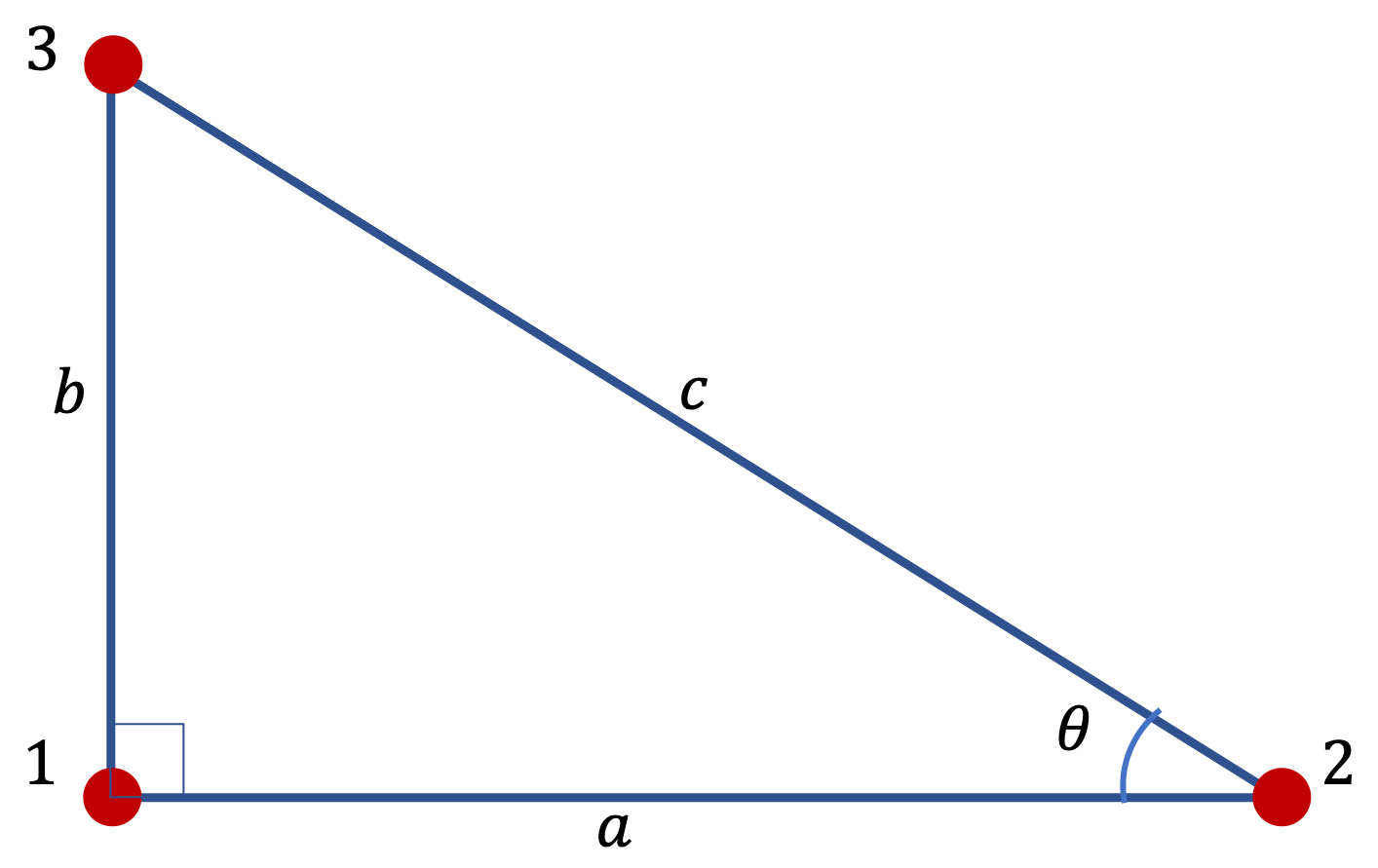(a) What are the magnitude and direction of the net gravitational force on 1 by 2 and 3?

(b) What are the magnitude and direction of the net gravitational force on 2 by 1 and 3?

(c) What are the magnitude and direction of the net gravitational force on 3 by 1 and 2?

Hint

(a), (b), (c): Find magnitudes of the forces and use components to add the vectors to obtain the net force.

(a) $10.5\times 10^{-7}\text{ N}$ at $72^\circ$ counterclockwise from positive $x$ axis. (b) $7.1\times 10^{-7}\text{ N}$ at $14.6^\circ$ clockwise from negative $x$ axis. (c) $12.2\times 10^{-7}\text{ N}$ at $73^\circ$ clockwise from positive $x$ axis.

Solution 1 (a)

(a) We first find the forces on 1 by 2 and 3 separately and then add them vectorially to obtain the net force on 1. Lets denote the magnitudes of these forces as $F_{21}$ and $F_{31}\text{.}$ The magnitudes of these forces are

\begin{align*} F_{21} \amp = G_N\dfrac{m_1 m_2}{a^2} \\ \amp = 6.67\times 10^{-11}\dfrac{200\times400}{4^2} = 3.3\times 10^{-7}\text{ N}. \\ F_{31} \amp = G_N\dfrac{m_1 m_3}{b^2} \\ \amp = 6.67\times 10^{-11}\dfrac{200\times300}{2^2} =10.0\times 10^{-7}\text{ N}. \end{align*}

Looks like we should use the unit of $10^{-7}\text{ N} \text{.}$ We will call this unit $\text{N*}$ to save space.

To add these forces, we will use analytic method. For that we inroduce axes. $F_{21}$ is towards positive $x$axis and $F_{31}$ towards positive $y$axis. From the figure we get

\begin{align*} F_\text{net} \amp = \sqrt{ F_{21}^2 + F_{31}^2 }\\ \amp = \sqrt{3.3^2 + 10.0^2} = 10.5 \text{ N*}\\ \amp = 10.5\times 10^{-7}\text{ N}. \end{align*}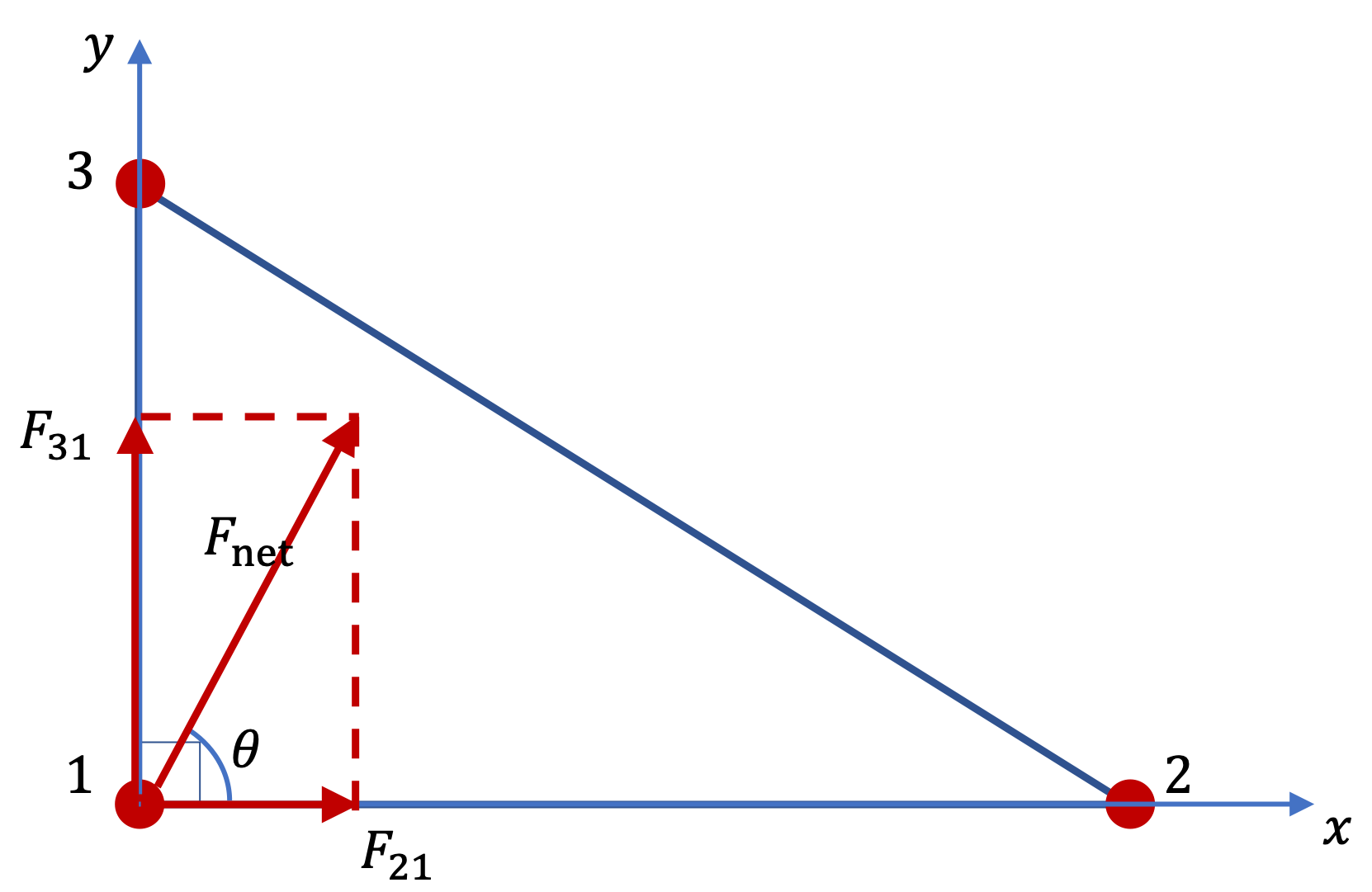We use angle $\theta$ to indicate the direction. The counterclockwise angle from the positive $x$ axis is

\begin{equation*} \theta = \tan^{-1}\left(\dfrac{10.0}{3.3} \right) = 72^\circ. \end{equation*}
Solution 2 (b)

Lets denote the magnitudes of forces on 2 by $F_{12}$ and $F_{32} \text{.}$ We have already worked the magnitude of the force between 1 and 2, which we had called $F_{21}\text{.}$

\begin{equation*} F_{12} = F_{21} = 3.3 \text{ N*}. \end{equation*}

The other force on 2 is

\begin{align*} F_{32} \amp = G_N\dfrac{m_2 m_3}{c^2} \\ \amp = 6.67\times 10^{-11}\dfrac{400\times300}{2^2 + 4^2} = 4.0\times 10^{-7}\text{ N}. \end{align*}

That is $F_{32} = 4.0\text{ N*}\text{.}$ To add these two forces we use the components.

\begin{align*} F_{12,x} \amp = -3.3\text{ N*}, \\ F_{12,y} \amp = 0, \\ F_{32,x} \amp = -4.0\times \dfrac{4}{\sqrt{2^2+4^2}} = -3.6\text{ N*}, \\ F_{32,y} \amp = 4.0\times \dfrac{2}{\sqrt{2^2+4^2}} = 1.8\text{ N*}, \end{align*}

Therefore, the components of the net force are

\begin{gather*} F_{\text{net},x} = -3.3 -3.6 = -6.9\text{ N*}, \\ F_{\text{net},y} = 0 + 1.8 = + 1.8\text{ N*}. \end{gather*}

The magnitude of this force is

\begin{equation*} F_{\text{net}} = \sqrt{ 6.9^2 + 1.8^2 } = 7.1\text{ N*} = 7.1\times 10^{-7}\text{ N}. \end{equation*}

The direction is in the second quadrant with clockwise angle with respect to the negative $x$ axis give by

\begin{equation*} \theta = \tan^{-1}\left(\dfrac{1.8}{6.9} \right) = 14.6^\circ. \end{equation*}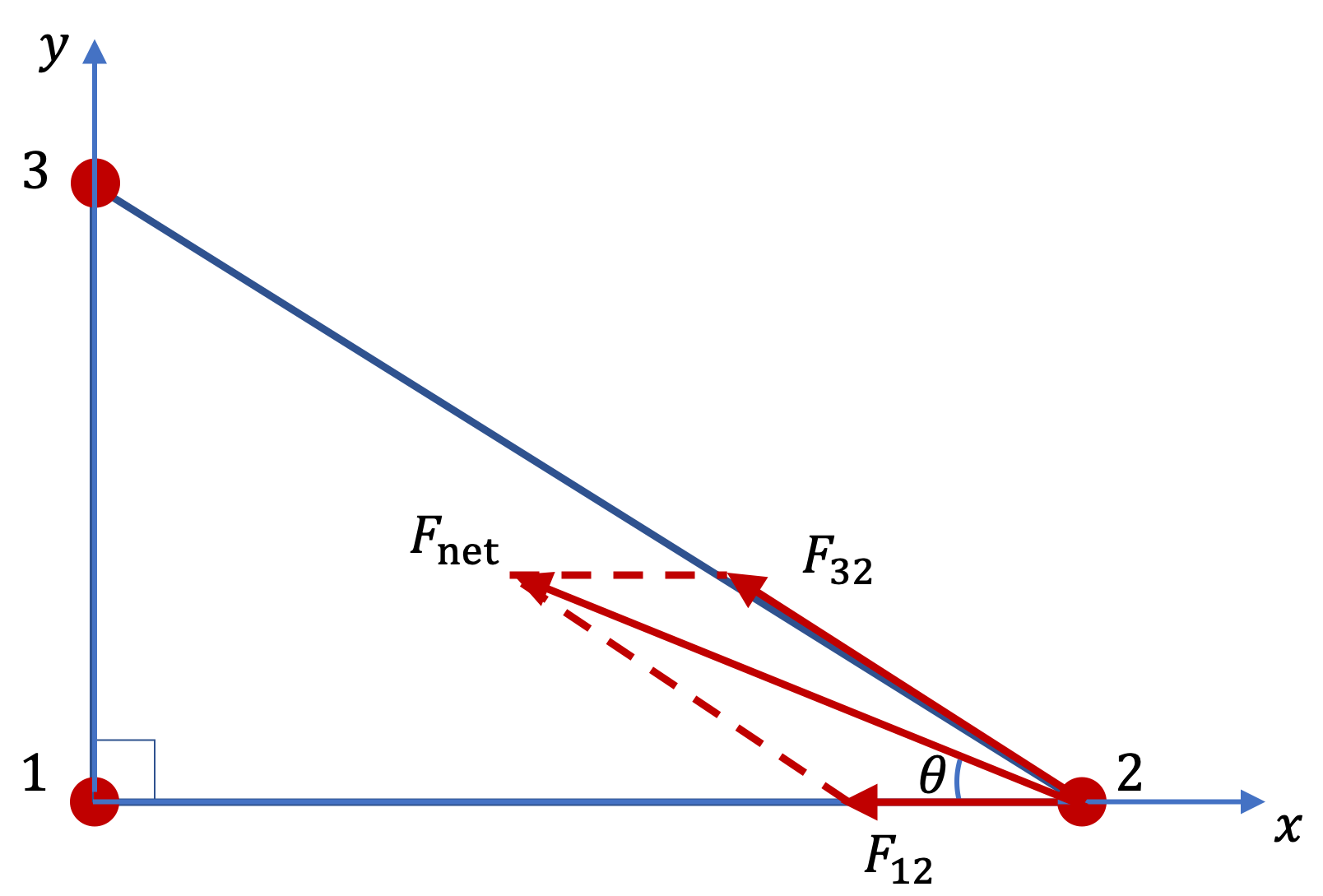Solution 3 (c)

(c) We have worked out the magnitudes $F_{13}$ and $F_{23}$ already as $F_{31}$ and $F_{32}\text{.}$

\begin{align*} F_{13} \amp = F_{31} = 10.0 \text{ N*}. \\ F_{23} \amp = F_{32} = 4.0 \text{ N*}. \end{align*}

We also know their components as per Newton's third law.

\begin{align*} F_{13,x} \amp = 0, \\ F_{13,y} \amp = -10.0\text{ N*}, \\ F_{23,x} \amp = - F_{32,x} = 3.6\text{ N*}, \\ F_{23,y} \amp = - F_{32,y} =-1.8\text{ N*}, \end{align*}

Therefore, the net force on 3 has the following components.

\begin{gather*} F_{\text{net},x} = 0 + 3.6 = 3.6 \text{ N*}, \\ F_{\text{net},y} = -10.0 - 1.8 = - 11.8\text{ N*}. \end{gather*}

The magnitude of this force is

\begin{equation*} F_{\text{net}} = \sqrt{ 3.^2 + 11.8^2 } = 12.2\text{ N*} = 12.2\times 10^{-7}\text{ N}. \end{equation*}

The direction is in the fourth quadrant with clockwise angle with respect to the positive $x$ axis give by

\begin{equation*} \theta = \tan^{-1}\left(\dfrac{11.8}{3.6} \right) = 73^\circ. \end{equation*}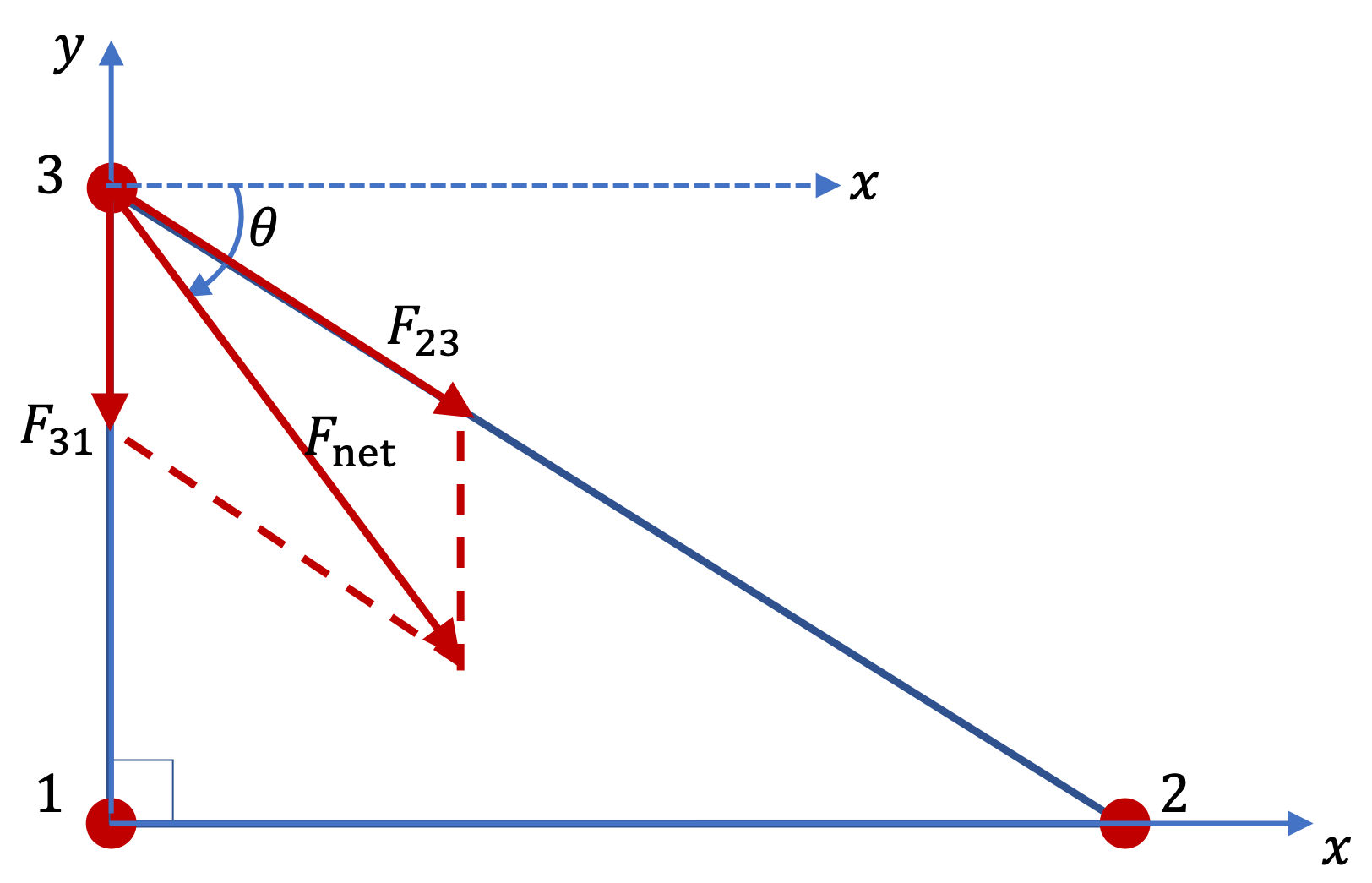A spherical ball of mass $m$ and radius $R$ is at one end of a uniform thin rod of mass $M$ and length $L$ as shown in Figure 12.2.8. What is the magnitude of the gravitational force between them?

Hint

Break up the rod into infinitesimal elements and set up an integral.

$G_N \dfrac{mM}{R(R+L)}$

Solution

We place the sphere with its center at the origin and the rod along $x$ axis as shown in Figure 12.2.9. Now consider the force on the sphere by an infinitesimal element of the rod between $x$ and $x+dx\text{.}$ The mass in the element $dx=\lambda dx\text{,}$ where $\lambda = M/L\text{,}$ the linear mass density.

\begin{equation*} dF = G_N\dfrac{1}{x^2}\, m\,\dfrac{M}{L} dx. \end{equation*}

We need to integrate this from $x=R$ to $x=R+L\text{.}$

\begin{align*} F \amp = G_N \dfrac{mM}{L}\, \int_{R}^{R+L}\dfrac{1}{x^2}\, dx, \\ \amp = G_N \dfrac{mM}{L}\, \left(\dfrac{1}{R} - \dfrac{1}{R+L}\right).\\ \amp = G_N \dfrac{mM}{R(R+L)}. \end{align*}

A spherical ball of mass $m$ and radius $R$ is at above the middle of a uniform thin rod of mass $M$ and length $L$ as shown in Figure 12.2.11. What is the magnitude of the gravitational force between them and direction of the force on the sphere?

Hint

Due to symmetry only the vertical component is non-zero.

Magnitude $\dfrac{ G_N m M }{ D \sqrt{D^2 + L^2/4} }$ and direction towards the center of the rod.

Solution

We place the mass of the sphere at its center and examine the force on this mass by two infintesimal elements of rod symmetrically placed as shown in Figure 12.2.12. Note that the direction of the two forces is such that the sum of their horizontal components is zero. Therefore, we need to find only the vertical component.

The $y$ component of force on the sphere by a small element between $x$ and $x + dx$ is

\begin{equation*} dF_y = -G_N \dfrac{m\, dM}{x^2 + D^2}\, \dfrac{D}{ \sqrt{ x^2 + D^2 } }. \end{equation*}

The infinitesimal mass, $dM = (M/L)dx$ .We integrate this from $x = -L/2$ to $x = L/2\text{.}$

\begin{align*} F_y \amp = -\int_{\text{rod}} G_N \dfrac{m\, dM}{x^2 + D^2}\, \dfrac{D}{ \sqrt{ x^2 + D^2 } },\\ \amp = -\dfrac{G_N m M D}{L} \int_{-L/2}^{L/2} \dfrac{1}{ \left( x^2 + D^2 \right)^{3/2} }\,dx,\\ \amp = -\dfrac{2G_N m M D}{L} \int_{0}^{L/2} \dfrac{1}{ \left( x^2 + D^2 \right)^{3/2} }\,dx,\\ \amp = -\dfrac{2G_N m M D}{L} \dfrac{ L/2 }{ D^2 \sqrt{D^2 + L^2/4} },\\ \amp = -G_N \dfrac{ m M }{ D \sqrt{D^2 + L^2/4} }. \end{align*}

Therefore, the force on the sphere has magnitude $\dfrac{ G_N m M }{ D \sqrt{D^2 + L^2/4} }$ and direction towards the center of the rod.

There is a small sphere of mass $m$ at a height $D$ from the center of a uniform ring of radius $R$ and mass $M\text{.}$ Find the gravitational force on the sphere.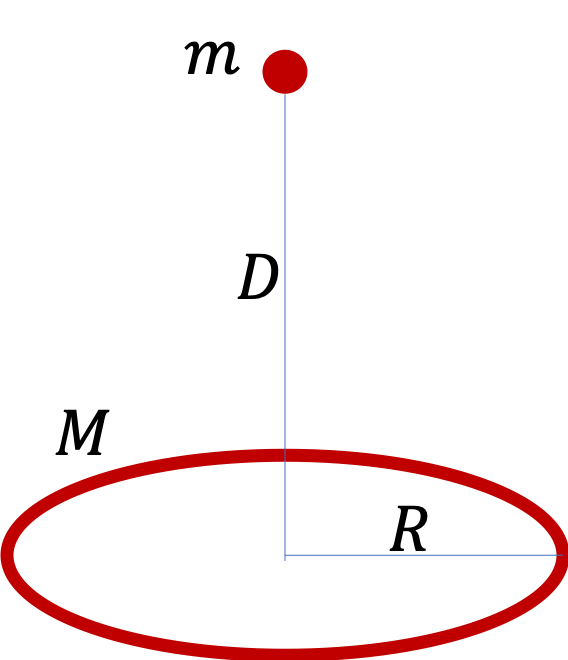Hint

Only vertical component is non-zero.

$\dfrac{G_N m M D}{ \left( D^2 + R^2 \right)^{3/2} }$ towards the center of the ring.

Solution

Looking at force from two elements of the ring that are at the diagonally opposite sides shows us that only the vertical component is non-zero. The magnitude of the force from an element of the ring on the sphere is

\begin{equation*} dF = G_N \dfrac{m\, (M/L) dl}{ D^2 + R^2} \end{equation*}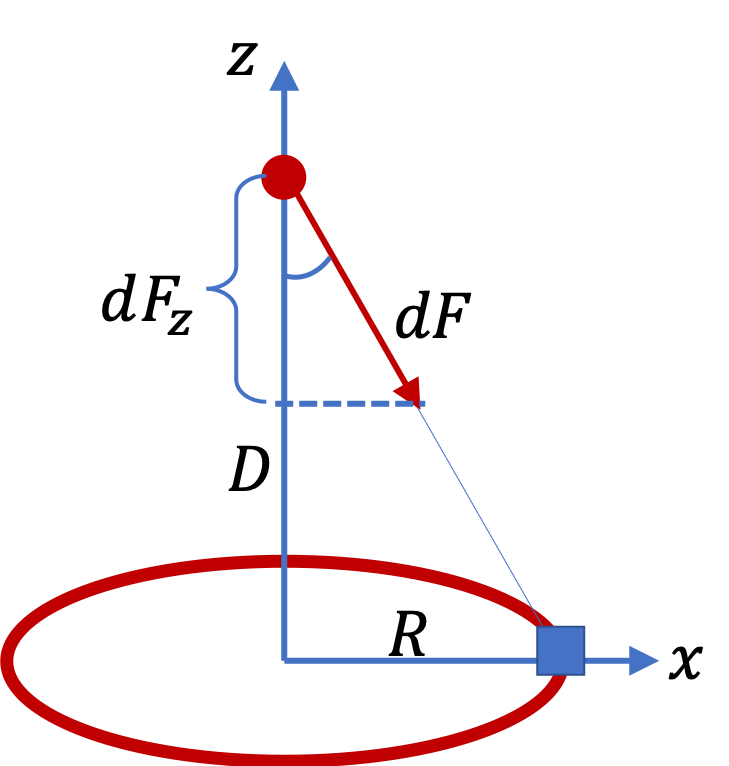Let $z$ axis be pointed up. Then, $z$ component of the gravitational force from an element of the ring of size $dl$ is

\begin{equation*} dF_z = - dF\, \dfrac{D}{ \sqrt{D^2 + R^2} }, \end{equation*}

which is independent of the location of the element on the ring. When we integrate this aroiund the ring, we will get

\begin{equation*} F_z = - G_N \dfrac{m M D}{ \left( D^2 + R^2 \right)^{3/2} }. \end{equation*}

Therefore, the magnitude of the force on the sphere is $\dfrac{G_N m M D}{ \left( D^2 + R^2 \right)^{3/2} }$ towards the center of the ring.

There is a small sphere of mass $m$ at a height $D$ from the center of a uniform disk of radius $R$ and mass $M\text{.}$ Find the gravitational force on the sphere.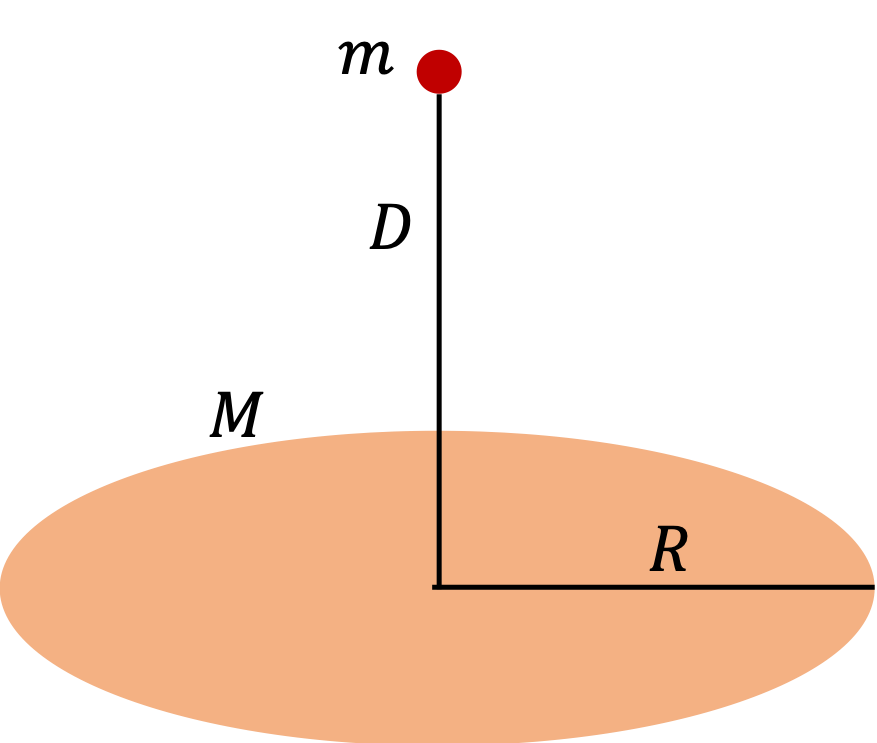Hint

Build the solution from the solution of a ring between $r$ and $r+dr\text{.}$

$\dfrac{2 G_N m M D}{R^2} \left( \dfrac{1}{D} - \dfrac{1}{\sqrt{D^2 + R^2}} \right)$ towards the center of the ring.

Solution

We will build the solution of the disk from the solution of a ring between $r$ and $r+dr\text{.}$ The mass in the infinitesimal ring will be

\begin{equation*} dM = \dfrac{M}{\pi R^2}\, 2\pi r dr, \end{equation*}

since area of the ring is $2\pi r dr\text{.}$ From symmetry, we also know that only the vertical component of the force is non-zero. Let $dF_z$ denote the $z$ component of the ring. From the ring solution we have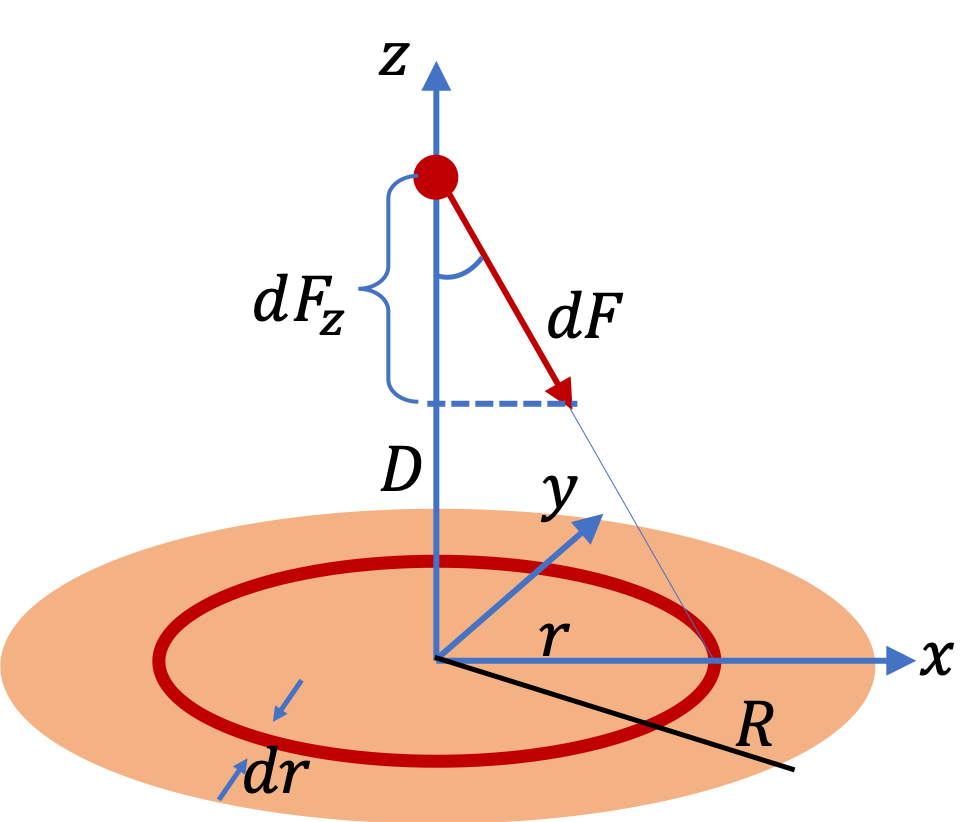\begin{align*} dF_z \amp = - G_N \dfrac{m\,D\,dM}{ \left( D^2 + r^2 \right)^{3/2} } \\ \amp = - G_N \dfrac{m\,D}{ \left( D^2 + r^2 \right)^{3/2} } \dfrac{M}{\pi R^2}\, 2\pi r dr\\ \amp = - \dfrac{G_N m M D}{R^2} \ \dfrac{2 r dr}{ \left( D^2 + r^2 \right)^{3/2} } \end{align*}

Now, we integrate this from $r=0$ to $r=R$ to get the contribution of the entire disk.

\begin{align*} F_z \amp = - \dfrac{G_N m M D}{R^2} \int_0^R \dfrac{2 r dr}{ \left( D^2 + r^2 \right)^{3/2} } \\ \amp = - \dfrac{G_N m M D}{R^2} \int_{D^2}^{D^2 + R^2} \dfrac{dy}{ y^{3/2} } \\ \amp = - \dfrac{2 G_N m M D}{R^2} \left( \dfrac{1}{D} - \dfrac{1}{\sqrt{D^2 + R^2}} \right) \end{align*}

The magnitude is the above quantity without the sign and direction is towards the center of the ring.